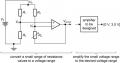# Desiging a circuit for a strain gauge.

Thread Starter

#### NMC

Joined Nov 16, 2018
13
Hi All,

Struggling with a design question and how to get started with it.

A strain gauge sensor, R4, has a resistance value from 110 ohms (no deflection) to 110.7 (full deflection). I need to design a circuit that will use this sensor to produce a voltage in the range [0.0V, 3.0V].

I need to calculate the value of Vsensor in the circuit.
Let R = R1 = R2 = R3, prove that Vsensor = Vs x (R-R4)/2R.I am struggling to see what voltage I would give Vs.
Any help would be appreciated.

Nath.

#### ericgibbs

Joined Jan 29, 2010
12,200
hi NMC,
Welcome to AAC.
Where did you get that circuit.?
E

EDIT:

This reads like a college assignment, moved to Homework Forum.

Last edited:

#### MrChips

Joined Oct 2, 2009
23,054
Here is an example of a strain gauge circuit.#### AlbertHall

Joined Jun 4, 2014
11,098
This may be a good strain gauge amplifier circuit but it doesn't offer TS much help with his calculation.

#### MrChips

Joined Oct 2, 2009
23,054
Presumable TS needs 3V FS for digitization with ADC. Adjust amplifier gain to suit. Do ADC and perform calibration in software.

#### AlbertHall

Joined Jun 4, 2014
11,098
I am struggling to see what voltage I would give Vs.
Any help would be appreciated.
You should be able to calcuate Va?
Va is the voltage on the non-inverting input of the opamp.
Next, the opamp has negative feedback and so will set its output to keep the inputs at the same voltage.
So what will be the voltage at VB?
Now you can calculate the voltage across R3 and so the current through R3.
Where does that current flow?

#### Porto

Joined Dec 11, 2018
4
Can you please be more specific?

#### ericgibbs

Joined Jan 29, 2010
12,200
hi Porto,
Welcome to AAC,
Who are you asking to be more specific.?E

#### Porto

Joined Dec 11, 2018
4
You should be able to calcuate Va?
Va is the voltage on the non-inverting input of the opamp.
Next, the opamp has negative feedback and so will set its output to keep the inputs at the same voltage.
So what will be the voltage at VB?
Now you can calculate the voltage across R3 and so the current through R3.
Where does that current flow?
How to calculate Va?
Va = Vs * R1/(R1+R2) ?
What will be the voltage at Vb?

MOD: use @AlbertHall as a Tag and he will be alerted.

Last edited by a moderator:

#### AlbertHall

Joined Jun 4, 2014
11,098
Your formula for Va is correct.
The amplifier has negative feedback and so Vb will be equal to Va.
https://www.electronics-tutorials.ws/opamp/opamp_2.html
Except:
The amplifier will have some input offset voltage - but for this question assume this is zero and perhaps state that assumption.
This will also only be true while the input and output voltages don't get too near the supply voltages. As no supply voltages are given again you can only assume that this will not be a problem.

#### Porto

Joined Dec 11, 2018
4
I'm still struggling with getting equation to work.

#### AlbertHall

Joined Jun 4, 2014
11,098
I'm still struggling with getting equation to work.
What problem do you have using the equation?
Do you know what values to use for each item in the equation?

#### Porto

Joined Dec 11, 2018
4
What problem do you have using the equation?
Do you know what values to use for each item in the equation?
I still don't know how to prove that Vsensor = Vs x (R-R4)/2R.

#### AlbertHall

Joined Jun 4, 2014
11,098
You can write an equation for the R3, R4 potential divider with Vs at the top, Vb in the middle and Vsensor at the bottom. Rearrange that equation with Vsensor as the subject (Vsensor = …………). You have a n equation for Vb so substitute that equation for Vb in your Vsensor equation and simplify the result.